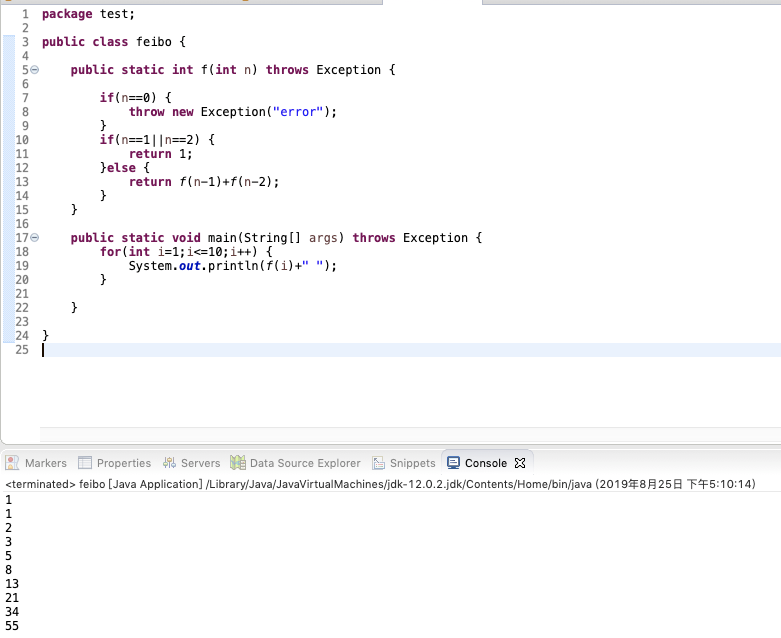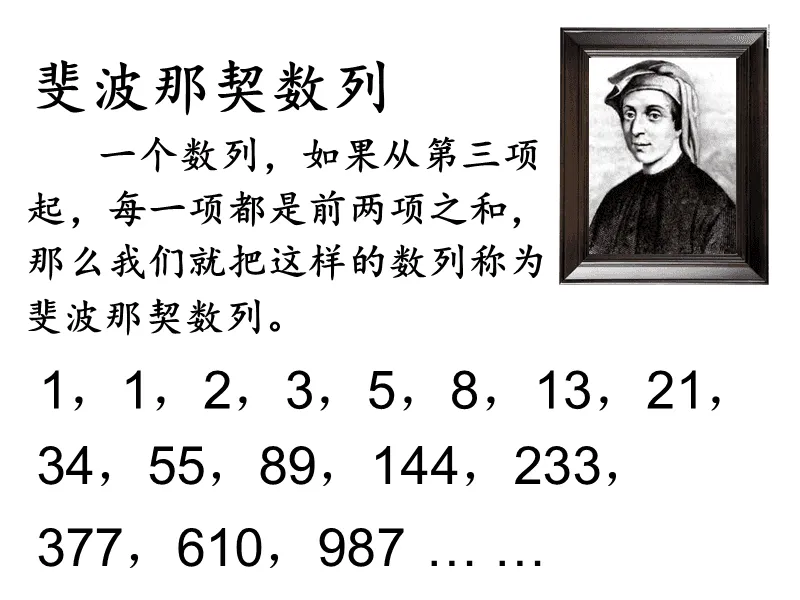• 2022-04-08 21:20:11

在不使用递归和公式法的情况下，求斐波那契数列，使用递推法，极大降低时间复杂度

public class Fei {

public static void main(String[] args) {
// TODO Auto-generated method stub
System.out.println("输出斐波那契的第20项/第20项，第一项是0，第二项为1");
int a = 0;
int b = 1;
int c = 1;
for(int i = 4; i <= 20; i++) {
a = b;
b = c;
c = a+b;
//System.out.print(" 第"+i+"项： "+c);
}
System.out.println();
System.out.println(c+"为答案");
}
}推荐算法
更多相关内容
• ## 斐波那契数列java代码实现

万次阅读 多人点赞 2021-09-09 23:43:39
斐波那契数列又称 黄金分割 数列，因数学家莱昂纳多·斐波那契（Leonardoda Fibonacci）以兔子繁殖为例子而引入，故又称为“ 兔子数列 ”; * 指的是这样一个数列：1、1、2、3、5、8、13、21、34、…… *其规律是从...

## 斐波那契数列

斐波那契数列又称 黄金分割 数列，因数学家莱昂纳多·斐波那契（Leonardoda Fibonacci）以兔子繁殖为例子而引入，故又称为“ 兔子数列 ”;

* 指的是这样一个数列：1、1、2、3、5、8、13、21、34、……
* 其规律是从第3个数开始，每个数都等于它前两个数的和。

## java代码实现

1. 用递归的方法实现:

package com;

import java.util.Arrays;

//1.递归方法
public class test2 {
//1.用递归方法
public static void main(String[] args) {
for (int counter = 0; counter <= 10; counter++){
System.out.printf("Fibonacci of %d is: %d\n", counter, fibonacci(counter));
}
}
public static long fibonacci(long number) {
if ((number == 0) || (number == 1))
return number;
else
return fibonacci(number - 1) + fibonacci(number - 2);
}
}

2. 用循环方法实现:

public class test3{
public static void main(String[] args) {
//1.定义三个变量,用来存放第一个第二第三个斐波那契数
int a1 = 1;
int a2 = 1;
int a3 ;
System.out.print("斐波那契数列前十个是:");
System.out.print(a1+","+a2);
for (int i = 3; i <=10;i++) {
//2.根据前两个数算出第三个数
a3=a2+a1;
//3.更新第一第二个数
a1=a2;
a2=a3;
System.out.print(","+a3);
}
}
}

3. 用数组+循环来实现:

public class test4{
public static void main(String[] args) {
//创建一个用来装斐波那契数列的数组,假设只获取前十个数
int [] arr = new int;
//先将前两个放入数组
arr = 1;
arr = 1;
//循环获得剩下斐波那契数
for (int i = 2; i <arr.length; i++) {
arr[i]=arr[i-1]+arr[i-2];
}
//输出这十个数
String s = Arrays.toString(arr);
System.out.println("前十个斐波那契数是:"+s);
}
}

展开全文算法
• 主要为大家详细介绍了Java递归实现斐波那契数列，具有一定的参考价值，感兴趣的小伙伴们可以参考一下
• 　斐波那契数列大家都非常熟悉。它的定义是： f(x) = 1 … (x=1,2) 　f(x) = f(x-1) + f(x-2) … (x>2) 对于给定的整数 n 和 m，我们希望求出： 　f(1) + f(2) + … + f(n) 的值。但这个值可能非常大，所以我们把它...
•展开全文• 主要介绍了java编程经典案例之基于斐波那契数列解决兔子问题,结合完整实例形式分析了斐波那契数列的原理及java解决兔子问题的相关操作技巧,需要的朋友可以参考下
• 主要为大家详细介绍了递归之斐波那契数列java的3种方法，具有一定的参考价值，感兴趣的小伙伴们可以参考一下
• 斐波那契数列：指的是这样的数列：0，1，1，2，3，5，8，21，34..., 这个数列从第三项开始，每一项都等于前两项之和。

斐波那契数列：指的是这样的数列：0，1，1，2，3，5，8，21，34..., 这个数列从第三项开始，每一项都等于前两项之和。案例代码：

/**
* 递归求解斐波那契数列
* 步骤解释：
* n=5 foo(4)+foo(3)
* =foo(3)+foo(2)+foo(2)+1
* =3foo(2) +1+1
* =3+2
* =5
*
* @param n
* @return
*/
private static Integer foo(int n) {
if (n < 2)
return n;
return foo(n - 1) + foo(n - 2);
}

循环、递归代码实现斐波那契数列

package top.chenxiky.courseware.sort;

/**
* Created with IntelliJ IDEA.
*
* @Author: chenxiky
* @Date: 2022/03/11/13:28
* @Description: 斐波那契数列
* 斐波那契数列：指的是这样的数列：0，1，1，2，3，5，8，21，34...,
* 这个数列从第三项开始，每一项都等于前两项之和
*/
public class FibonacciLoop {
public static void main(String[] args) {
// 笔试题
System.out.println("答案:" + foo(5));
// 循环实现斐波那契数列
fibonacci_loop(5);
// 打印空行
System.out.println();
// 递归实现斐波那契数列
fibonacci_recursion(1);
}

/**
* 递归求解斐波那契数列
* 步骤解释：
* n=5 foo(4)+foo(3)
* =foo(3)+foo(2)+foo(2)+1
* =3foo(2) +1+1
* =3+2
* =5
*
* @param n
* @return
*/
private static Integer foo(int n) {
if (n < 2)
return n;
return foo(n - 1) + foo(n - 2);
}

/**
* 用循环实现斐波那契数列
*
* @return
*/
public static void fibonacci_loop(int num) {

// num如果小于1直接结束该方法
if (num < 1) {
return;
}
// 设置一个变量输出数据的个数
int n = 0;
// 初始化第一个数
int a = 0;
// 初始化第二个数
int b = 1;
// 第三个数 斐波那契数列第一项等于前两项之和(初始化为0)
int c = 0;

// do while至少循环一次
do {
n++;
if (n == 1) {
System.out.print("循环实现：" + 0 + "\t");
System.out.print(1 + "\t");
}
// 第三个数是前两个数之和
c = a + b;
System.out.print(c + "\t");
// 原来的第一个数变为第二个数
a = b;
// 原来的第二个数变为第三个数
b = c;
} while (n < num);
return;
}

/**
* 递归实现斐波那契数列
*
* @param num
*/
public static void fibonacci_recursion(int num) {
// 边界值判断
if (num < 1) {
return;
}
// 调用递归方法
fibonacci_recursion_handler(1, num, 0, 1);

}

/**
* 在私有的方法里递归调用
*
* @param index // 数据的序号
* @param num   //  数列数据的个数
* @param one   //   第一个数
* @param two   //    第二个数
*/
private static void fibonacci_recursion_handler(int index, int num, int one, int two) {

// 程序的出口
if (index <= num) {
if (index == 1) {
System.out.print("递归实现：" + one + "\t");
System.out.print(two + "\t");
}
// 第三个数是前两个数之和
System.out.print(one + two + "\t");
// 递归 原来的第一个数变成第er个数 原来的第二个数变成第三个数
fibonacci_recursion_handler(++index, num, two, one + two);
}

//        // 程序的出口
//        if (index > num) {
//            // 终止递归
//            return;
//        } else {
//            if (index == 1) {
//                System.out.println(0);
//                System.out.println(1);
//            }
//            // 第三个数是前两个数之和
//            System.out.println(one + two);
//            // 原来的第一个数变成第er个数 原来的第二个数变成第三个数
//            fibonacci_recursion_handler(++index, num, two, one + two);
//        }

}

}


展开全文算法 idea
• 题目： 描述：斐波那契数列(Fibonacci sequence)，指的是这样一个数列：0、1、1、2、3、5、8、13、21、34...//采用递归的方法fibonacci数列 import java.util.Scanner ; public class fibonacci { public static void算法 动态规划 leetcode
• [斐波那契数列] 意大利著名文学家斐波那契在研究兔子繁殖问题时，发现有这样的一列数：1，1，2，3，5，8，.......；该数列的特点是：前两个数均为1，从第三个数起，每一个数都等于它前两个数的和，人们把这样的一组...算法
• Fibonacci数列的递推公式为：Fn=Fn-1+Fn-2，其中F1=F2=1。 当n比较大时，Fn也非常大，现在我们想知道，Fn除以10007的余数是多少。 输入格式 输入包含一个整数n。 输出格式 输出一行，包含一个整数，表示Fn除以...算法
• 参考：斐波那契数列为什么那么重要，所有关于数学的书几乎都会提到？ 快速幂 1.快速幂 快速幂算法的核心思想就是每一步都把指数分成两半，而相应的底数做平方运算。这样不仅能把非常大的指数给不断变小，所需要执行...
• 蓝桥杯 入门训练 Fibonacci数列 JAVA import java.util.Scanner; class Main{ public static void main(String[] args) { Scanner sc = new Scanner(System.in); int n =sc.nextInt(); if(n==1||n==2){ System.o
• 展开全部Java编程：三种方法实现斐波那契数列其一方法：publicclassDemo2{//定义三个变量方法publicstaticvoidmain(String[]args){inta=1,b=1,c=0;System.out.println("斐波那契数列前20项为：");System.out.print...
• /** * @author wufurun ... * @Description: 斐波那契数列 1 1 2 3 5 8 13 即 f(n) = f(n-1)+f(n-2) * @date 2021/4/414:12 */ public class Fibonacci { private static int data[] = new in...
• 斐波那契数列Fibonacci sequence），又称黄金分割数列、因数学家列昂纳多·斐波那契（Leonardoda Fibonacci）以兔子繁殖为例子而引入，故又称为“兔子数列”。java代码实现该数列
• 主要介绍了fibonacci数列(斐波那契数列)示例，大家参考使用吧
• 斐波那契数列fibonacci）-java的非递归实现。
• Fibonacci数列的递推公式为：Fn=Fn-1+Fn-2，其中F1=F2=1。 随机输入一个整数n，求得 Fn除以10086的余数是多少 输入格式: 输入一个整数n 输出格式: 输出一个整数Fn除以10086的余数 输入样例: 3 输出样例: 2...
• Fibonacci数列的递推公式为：Fn=Fn-1+Fn-2，其中F1=F2=1。 当n比较大时，F也非常大，现在我们想知道，Fn除以10007的余数是多少。 解题思路 最简单的方法应该是使用递归 代码如下 private static int Fibonacci(int n...算法
• @author：肖佳嘉 @Date：May 1, 2019 package File_Statistics; import java.math.BigInteger; public class File05_Fibonacci { ... //通过递归实现斐波那契数列的计算 //1 1 2 3 5 8 13 21 ...算法
•后端
• 数列：0,1,1,2,3,5,8,13… 其实就是每一个数都等于前面两位的和 简单思路就是递归，输入n，就是斐波那契的第n位的值，然后当递归到n时，递归结束，输出第n位的值。 代码： public static int n=2;//出门ab就有两位了...数据结构 leetcode 算法
• Fibonacci数列 {1,1,2,3,5,8,13,21,34......} ） ①用数组实现 public static void main(String[] args) { Scanner N = new Scanner(System.in); System.out.println("输入月份n:"); int n = N.nex
• BASIC-04 Fibonacci数列 问题描述 Fibonacci数列的递推公式为：Fn = Fn-1 + Fn-2，其中 F1 = F2 = 1。 当n比较大时，Fn 也非常大，现在我们想知道，Fn 除以 10007 的余数是多少。 输入格式 输入包含一个整数n。 ...
• 斐波那契数列Fibonacci sequence），又称黄金分割数列，因数学家莱昂纳多·斐波那契（Leonardo Fibonacci）以兔子繁殖为例子而引入，故又称为“兔子数列”，指的是这样一个数列：1、1、2、3、5、8、13、21、34、...
• 斐波那契数列(Fibonacci)JAVA解法 1.递归函数： public class Main { public int f(int n){ if (n == 0 | n==1) return 1; else return (f(n-1)+f(n-2)); } public static void main(String[] args) { ...算法
• //java编程：三种方法实现斐波那契数列 //其一方法： public class Demo2 { // 定义三个变量方法 public static void main(String[] args) { int a = 1, b = 1, c = 0; System.out.println("斐波那契...

# 斐波那契数列javajava 订阅